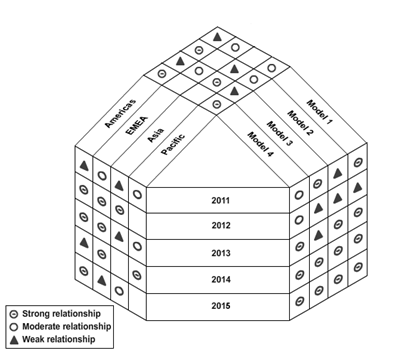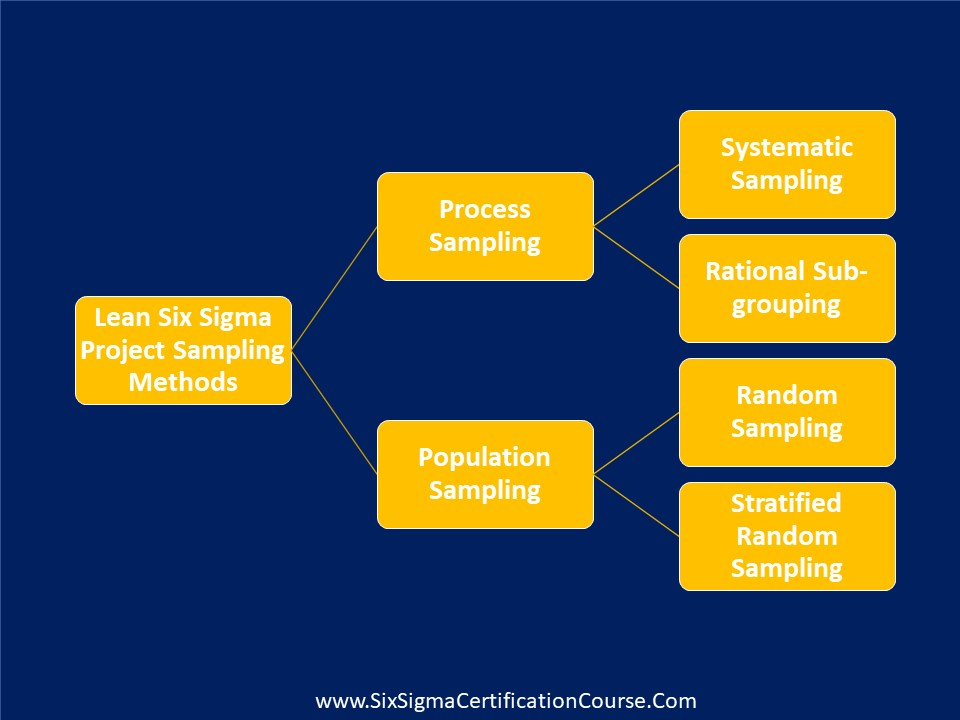# What is Y-Shaped Matrix Diagram?

The Y-shaped matrix is a matrix diagram that relates three sets of elements where one set is related to the other two sets in a circular manner. . It can be formed by bending the columns of sets A and B in the T-matrix in such a way that there is an interrelation between the elements of these two sets.Fig: A Y-shaped matrix that summarizes the requirements for different models of photocopiers

##### Related Articles##### Protected: Six Sigma Primer Quiz##### What do you understand by Theory of Constraints (ToC)?##### Selecting the Right Sampling Method for Lean Six Sigma Projects